# 一、直接插入的基本思想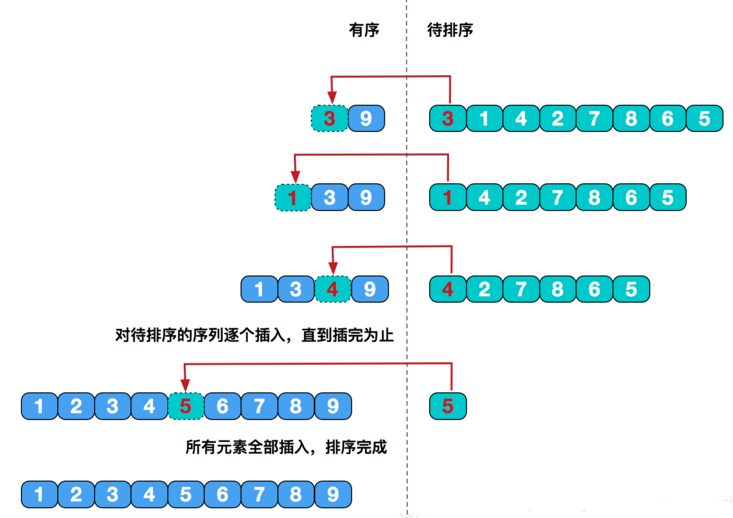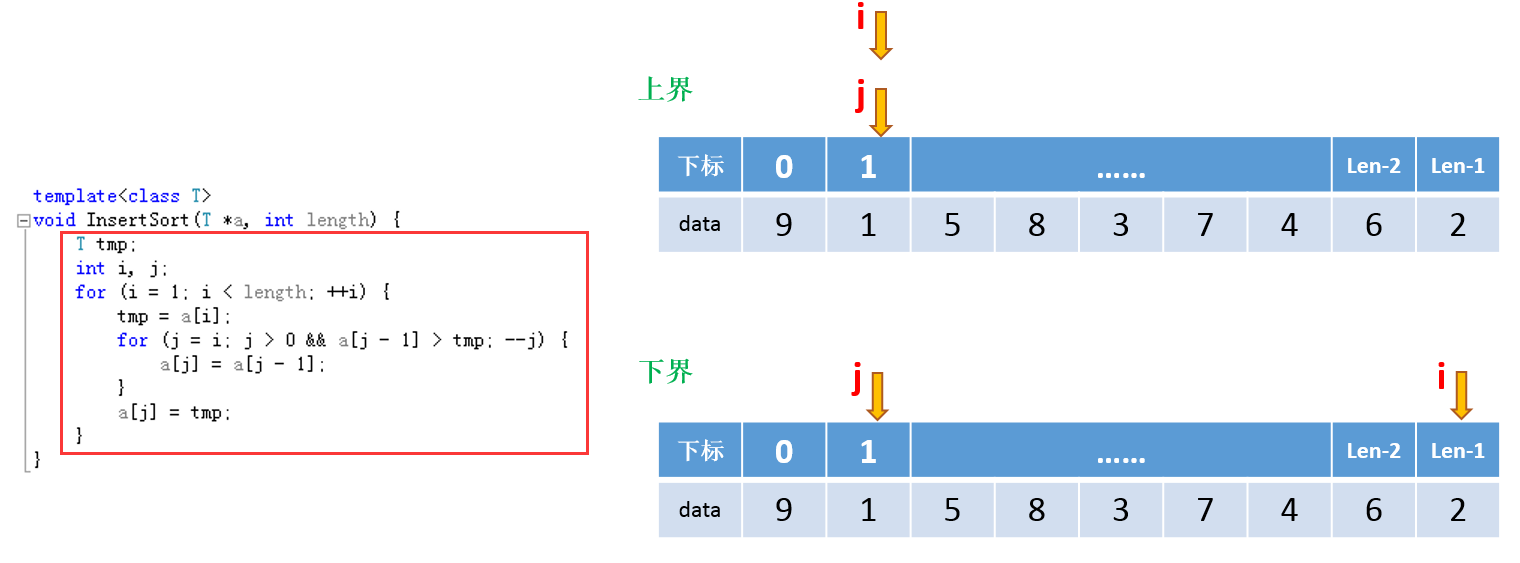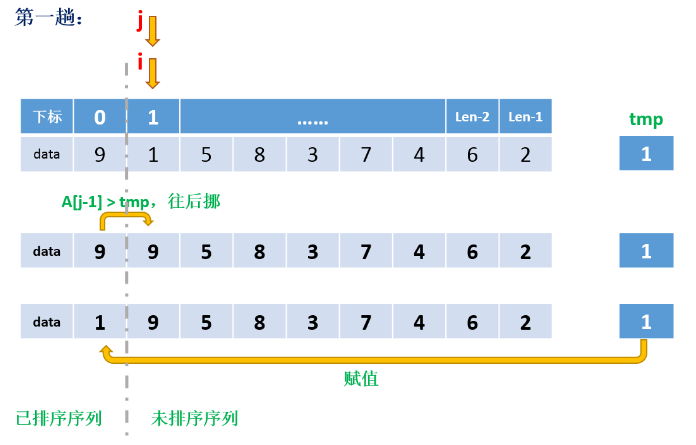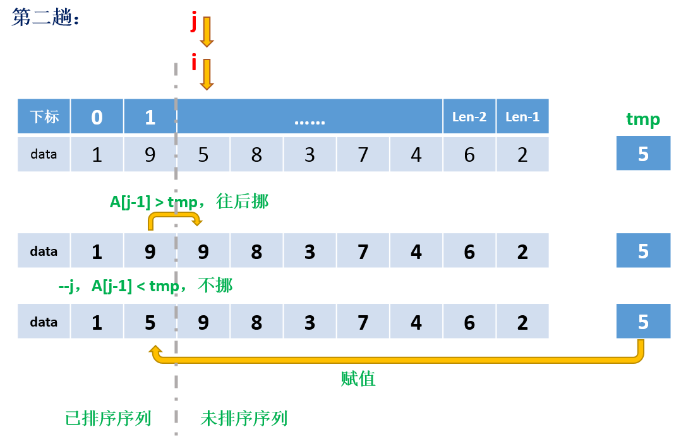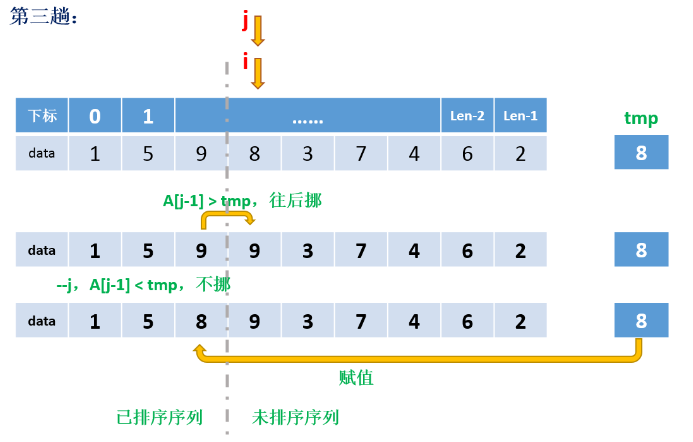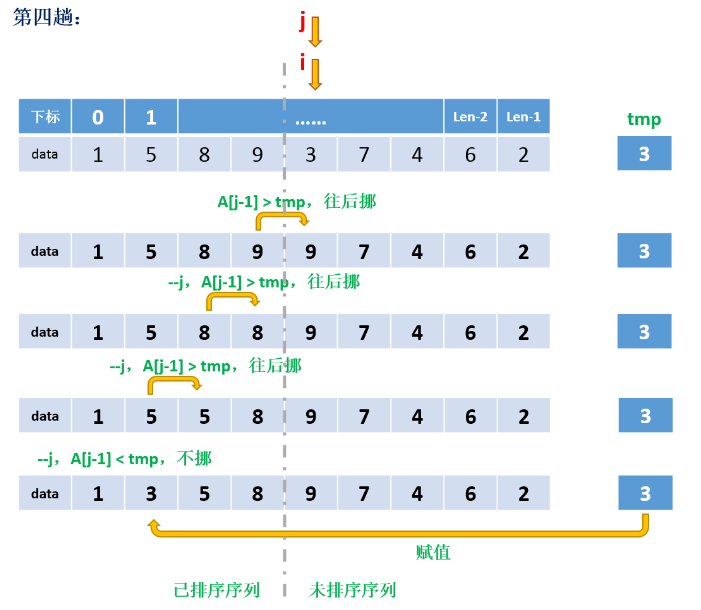# 二、折半（二分）插入排序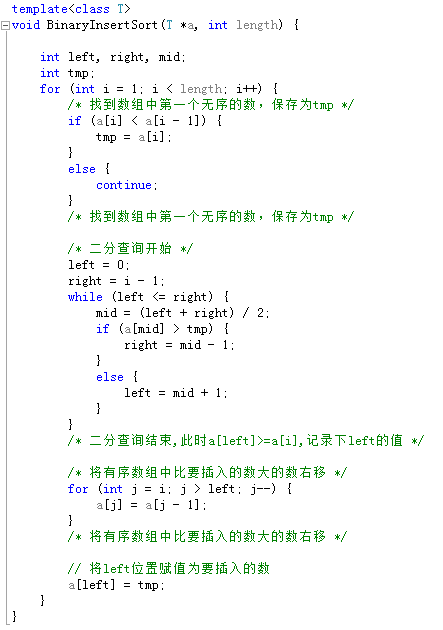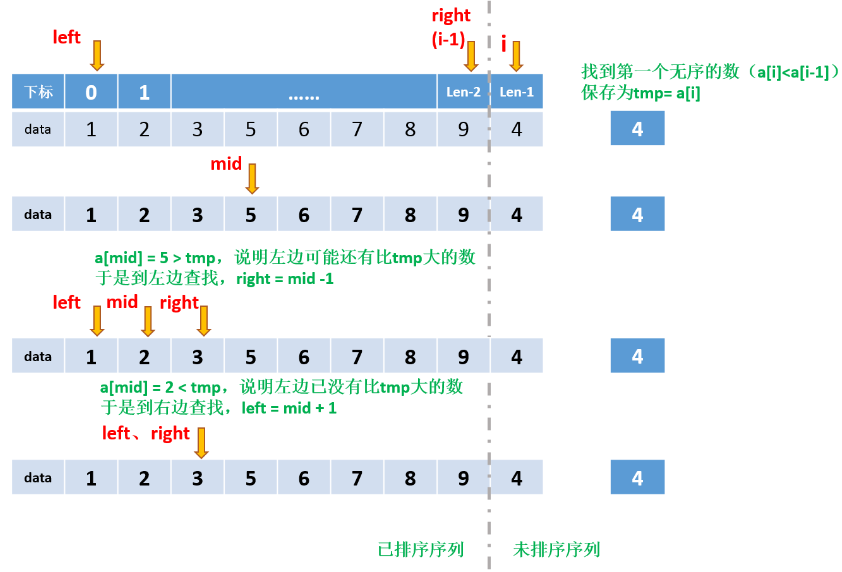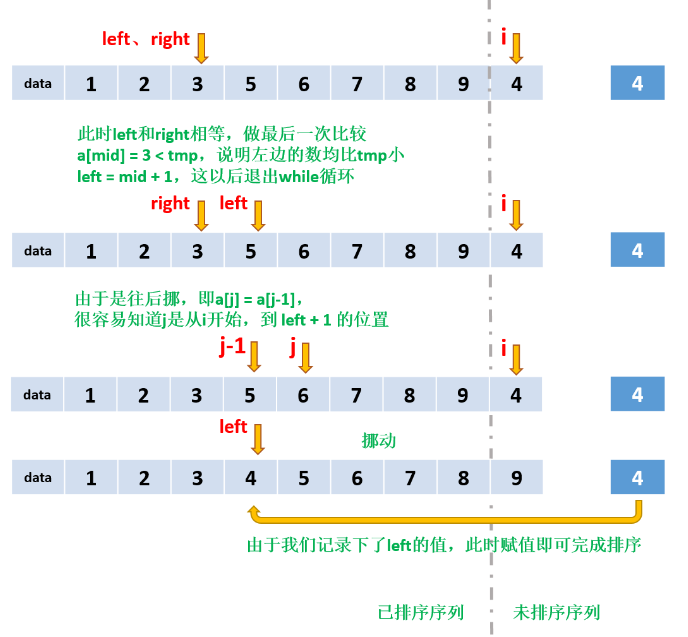# 三、測試結果及程式碼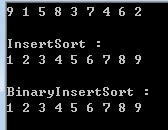``````#include <iostream>

template<class T>
void InsertSort(T *a, int length) {
T tmp;
int i, j;
for (i = 1; i < length; ++i) {
tmp = a[i];
for (j = i; j > 0 && a[j - 1] > tmp; --j) {
a[j] = a[j - 1];
}
a[j] = tmp;
}
}

template<class T>
void BinaryInsertSort(T *a, int length) {

int left, right, mid;
int tmp;
for (int i = 1; i < length; i++) {
/* 找到陣列中第一個無序的數，儲存為tmp */
if (a[i] < a[i - 1]) {
tmp = a[i];
}
else {
continue;
}
/* 找到陣列中第一個無序的數，儲存為tmp */

/* 二分查詢開始 */
left = 0;
right = i - 1;
while (left <= right) {
mid = (left + right) / 2;
if (a[mid] > tmp) {
right = mid - 1;
}
else {
left = mid + 1;
}
}
/* 二分查詢結束,此時a[left]>=a[i],記錄下left的值 */

/* 將有序陣列中比要插入的數大的數右移 */
for (int j = i; j > left; j--) {
a[j] = a[j - 1];
}
/* 將有序陣列中比要插入的數大的數右移 */

// 將left位置賦值為要插入的數
a[left] = tmp;
}
}

template<class T>
void ArrShow(T *a, int length) {
for (int i = 0; i < length; ++i) {
std::cout << a[i] << " ";
}
puts("\n");
}

int main(int argc, char *argv[]) {
int test = { 9, 1, 5, 8, 3, 7, 4, 6, 2 };
ArrShow(test, 9);

puts("InsertSort : ");
InsertSort(test, 9);
ArrShow(test, 9);

int test1 = { 9, 1, 5, 8, 3, 7, 4, 6, 2 };
puts("BinaryInsertSort : ");
BinaryInsertSort(test1, 9);
ArrShow(test1, 9);

return 0;
}
``````